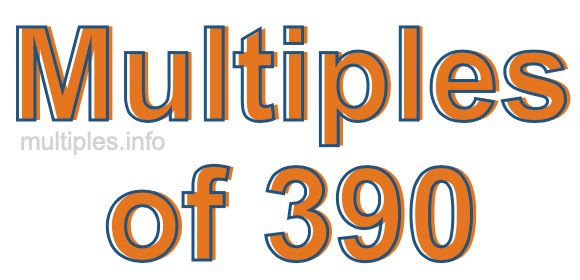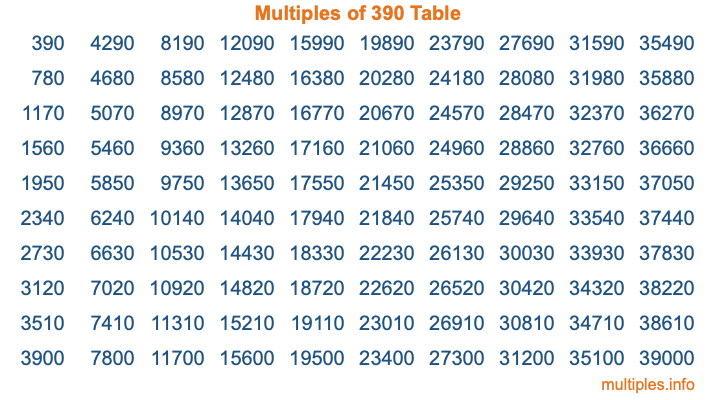Multiples of 390Welcome to the Multiples of 390 page. Here we will first teach you everything you will ever need to know about the multiples of 390, and then give you a study guide summary of everything we taught you to make sure you remember it all. Use this page to look up facts and learn information about the multiples of 390. This page will make you a multiples of three hundred ninety expert!

Definition of Multiples of 390
Multiples of 390 are all the numbers that when divided by 390 equal an integer. Each of the multiples of 390 are called a multiple. A multiple of 390 is created by multiplying 390 by an integer.

Therefore, to create a list of multiples of 390, you start with 1 multiplied by 390, then 2 multiplied by 390, then 3 multiplied by 390, and so on for as long as you want. Thus, the list of the first five multiples of 390 is 390, 780, 1170, 1560, and 1950. To see a larger list of multiples of 390, see the printable image of Multiples of 390 further down on this page. We also have a category where you can choose any nth multiple of 390.

Multiples of 390 Checker
The Multiples of 390 Checker below checks to see if any number of your choice is a multiple of 390. In other words, it checks to see if there is any number (integer) that when multiplied by 390 will equal your number. To do that, we divide your number by 390. If the the quotient is an integer, then your number is a multiple of 390.

Is  a multiple of 390?

Least Common Multiple of 390 and ...
A Least Common Multiple (LCM) is the lowest multiple that two or more numbers have in common. This is also called the smallest common multiple or lowest common multiple and is useful to know when you are adding our subtracting fractions. Enter one or more numbers below (390 is already entered) to find the LCM.

Check out our LCM Calculator if you need more details about the Least Common Multiple or if you need the LCM for different numbers for adding and subtraction fractions.

nth Multiple of 390
As we stated above, 390 is the first multiple of 390, 780 is the second multiple of 390, 1170 is the third multiple of 390, and so on. Enter a number below to find the nth multiple of 390.

th multiple of 390

Multiples of 390 vs Factors of 390
390 is a multiple of 390 and a factor of 390, but that is where the similarities end. All postive multiples of 390 are 390 or greater than 390. All positive factors of 390 are 390 or less than 390.

Below is the beginning list of multiples of 390 and the factors of 390 so you can compare:

Multiples of 390: 390, 780, 1170, 1560, 1950, etc.

Factors of 390: 1, 2, 3, 5, 6, 10, 13, 15, 26, 30, 39, 65, 78, 130, 195, 390

As you can see, the multiples of 390 are all the numbers that you can divide by 390 to get a whole number. The factors of 390, on the other hand, are all the whole numbers that you can multiply by another whole number to get 390.

It's also interesting to note that if a number (x) is a factor of 390, then 390 will also be a multiple of that number (x).

Multiples of 390 vs Divisors of 390
The divisors of 390 are all the integers that 390 can be divided by evenly. Below is a list of the divisors of 390.

Divisors of 390: 1, 2, 3, 5, 6, 10, 13, 15, 26, 30, 39, 65, 78, 130, 195, 390

The interesting thing to note here is that if you take any multiple of 390 and divide it by a divisor of 390, you will see that the quotient is an integer.

Multiples of 390 Table
Below is an image of the first 100 multiples of 390 in a table. The table is in chronological order, column by column. The first column has the first ten multiples of 390, the second column has the next ten multiples of 390, and so on.The Multiples of 390 Table is also referred to as the 390 Times Table or Times Table of 390. You are welcome to print out our table for your studies.

Negative Multiples of 390
Although not often discussed or needed in math, it is worth mentioning that you can make a list of negative multiples of 390 by multiplying 390 by -1, then by -2, then by -3, and so on, to get the following list of negative multiples of 390:

-390, -780, -1170, -1560, -1950, etc.

Multiples of 390 Summary
Below is a summary of important Multiples of 390 facts that we have discussed on this page. To retain the knowledge on this page, we recommend that you read through the summary and explain to yourself or a study partner why they hold true.

There are an infinite number of multiples of 390.

A multiple of 390 divided by 390 will equal a whole number.

390 divided by a factor of 390 equals a divisor of 390.

The nth multiple of 390 is n times 390.

The largest factor of 390 is equal to the first positive multiple of 390.

390 is a multiple of every factor of 390.

390 is a multiple of 390.

A multiple of 390 divided by a divisor of 390 equals an integer.

390 divided by a divisor of 390 equals a factor of 390.

Any integer times 390 will equal a multiple of 390.

Multiples of a Number
Here you can get the multiples of another number, all with the same attention to detail as we did for multiples of 390 on this page.

Multiples of
Multiples of 391
Did you find our page about multiples of three hundred ninety educational? Do you want more knowledge? Check out the multiples of the next number on our list!